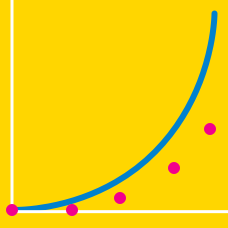Quantitative Finance

Root Approximation - Bisection

The equation $\displaystyle e^x = \frac{1}{x}$ has a solution somewhere between $0$ and $1.$ Equivalently, we seek a root of the continuous function $x e^{x}-1$ in the interval $(0,1).$ If we apply the bisecton method 4 times, which of the following intervals will we end up with?

One of the roots of the equation $\displaystyle f(x)=3x+\sin x-e^x = 0$ lies between $0$ and $0.5.$ If we apply the bisecton method 5 times, which of the following intervals will we end up with?

Determine the value of $\displaystyle \sqrt{5}$ by using the bisecton method. Let the width of the final interval less than $0.02,$ and start with the interval $[1,2].$ Then, guess the upper boundary of the final interval as the value of $\displaystyle \sqrt{5}.$

The equation $\displaystyle (x-2)^3+(x-2)^2-1=0$ has a root between $2$ and $3.$ If we apply the bisecton method 6 times, which of the following intervals will we end up with?

The equation $\displaystyle 2^x+2^{-x} = 3$ has a root between $1$ and $2.$ If we apply the bisecton method 5 times, which of the following intervals will we end up with?

×